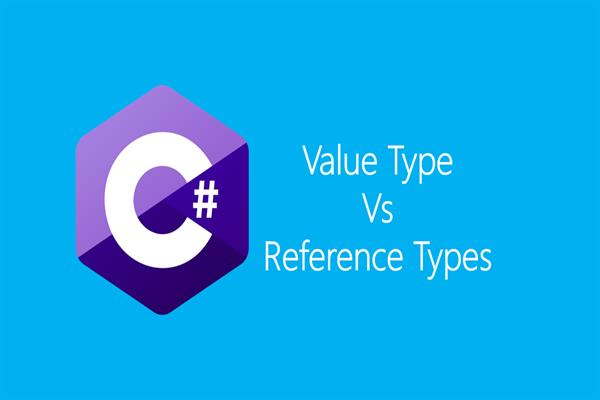Loading, please wait...# Differentiate between value type and reference data types with example.

Jun 14, 2020 valuetype, referencetype, 3506 Views
In this article you will learn about difference between value type and reference data types with example.

Value Type Vs Reference Types

A key difference between a value type and a reference type is the memory location associated with the type with a value type variable, it contains the actual value of the variable, such as 3.1416 or 343.

By contrast, the memory location associated with a reference type variable contains an address (called a pointer) that indicates where the actual object is in memory.

A local variable of value type are stored always on the stack. A stack is a data structure that stores items in a first-in-first-out (FIFO) fashion. It is an area of memory supported by the processor and its size is determined at the compile time.

By contrast, variables of reference types are always allocated on heap memory. A heap consists of memory available to the program at runtime. The allotment is done dynamically during the execution time.

While assigning or copying in a value type, a variable value is copied by creating a separate copy of the variable in memory. Therefore, an assignment operation results in two separate, but identical of the value in memory.

Example:

``````int x=100;

// variable x is holding integer value 100 x memory=100

int y=x;

// x variable value is assigned to y variable y memory=100``````

Now both x and y variables will have values each 100.

By contrast assignment statement between two reference variables result in two references to a single value at one location as shown through the following example:

``````string k1=”sum”;

// variable k1 acts as a pointer to “sum” string object

string k2=k1;

// now both k1 and k2 refer to “sum”``````

When value types are passed to methods (or procedures) as arguments, the exact copy of the value is passed.

Example:

``````// Illustrate of value types passing to methods.

Using system;
namespace chapter01
{
public class ValueTypes
{
public void swap(int x, int y)
{
int temp=0;
temp=x;
x=y;
y=temp;
}
}

public static void Main()
{
int a=400, b=700;
ValueTypes vt=new ValueTypes();
Console.WriteLine(“before swapping a={0},b={1}”,a,b);
vt.swap(a, b);
Console. Writeline(“after swapping a={0},b={1}”,a,b);
}
}``````

Output:

Before swapping a=400, b=700

after swapping a=400, b=700

By contrast when reference types are passed to methods (or procedures) as a parameter, then only a reference to the object is copied and not the actual contents.

Example:

``````// Illustrate of reference types passing to methods.

Using System;
namespace Chapter01
{
public class Reference Types
{
public void swap(ref int x, ref int y)
{
int temp=0;
temp=x;
x=y;
y=temp;
}
}

public static void Main()
{
int a=400, b=700;
ValueTypes vt=new ValueTypes();
Console.WriteLine(“before swapping a={0},b={1}”,a,b);
vt.swap(ref a, ref b);
Console. Writeline(“after swapping a={0},b={1}”,a,b);
}
}``````

Output:

Before swapping a=400, b=700

after swapping a=700, b=400

The value types when not initialized explicitly gets initialized explicitly, gets initialized with 0 in case of numbers, false in case of bool type, and by value ‘\0’ in case of char.

• By contrast, when an object is not initialized by default it refers to what is known as null.
• The value types are disposed of once they leave scope.
• The disposal of reference types is done automatically by a method known as a garbage collector.